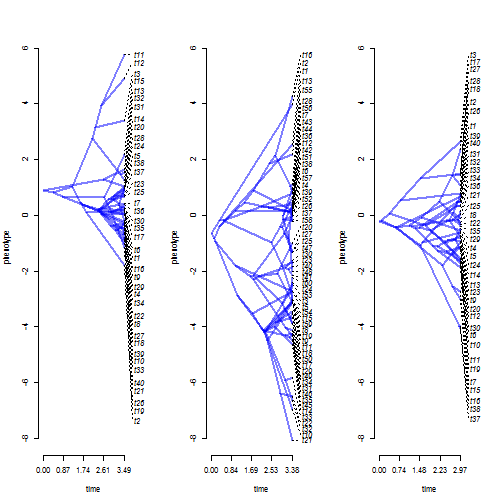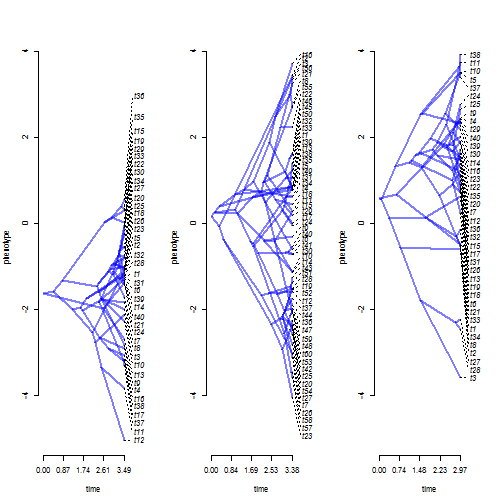## Monday, September 18, 2017

### `ratebytree` with posthoc tests

I just pushed a pretty substantive update to the phytools function `ratebytree` for comparing rates (and processes) of evolutionary change among trees. The method essentially extends the 'censored' model of O'Meara et al. (2006), but applied to the problem of comparing between different trees and modeling processes other than Brownian motion evolution of continuous traits.

What I had not included, to this point, was any posthoc comparison of rates (or evolutionary parameter estimates - for processes other than BM) between trees. Thus - for more than two trees, we might be able to tell that evolutionary rates are different, but perhaps not which ones differ significantly one from the other.

I have now fixed this in two ways. First, I obtain the Hessian (curvature) matrix from the likelihood surface around the MLE from which I could calculate variances and standard errors of the parameter estimates for each model. These are now output as part of the `"ratebytree"` object and printed by the S3 `print` method of the object class. Second, I developed a new method called `posthoc` which conducts a set of pairwise posthoc tests between each pair of trees and reports the t-statistics and P-values for each comparison. The user can optionally supply a value to the argument `p.adjust.method` to adjust the reported P-values due to multiple testing. (Alternatively, one could just use a stricter rejection threshold.)

Here I will demonstrate using some simulated trees & data.

``````library(phytools)
packageVersion("phytools")
``````
``````##  '0.6.26'
``````
``````print(trees,details=TRUE)
``````
``````## 3 phylogenetic trees
## tree 1 : 40 tips
## tree 2 : 60 tips
## tree 3 : 40 tips
``````
``````par(mfrow=c(1,3))
## x
nulo<-mapply(phenogram,tree=trees,x=x,
col=make.transparent("blue",0.5)))
``````
``````## Optimizing the positions of the tip labels...
``````
``````## Optimizing the positions of the tip labels...
``````
``````## Optimizing the positions of the tip labels...
````````````## y
nulo<-mapply(phenogram,tree=trees,x=y,
col=make.transparent("blue",0.5)))
``````
``````## Optimizing the positions of the tip labels...
``````
``````## Optimizing the positions of the tip labels...
``````
``````## Optimizing the positions of the tip labels...
``````Now, at a glance, it may be kind of hard to tell which character (if either) has evolved with a difference in rate between trees - and which has not. Luckily, we have a method to test this! Let's see what it shows.

``````fitBM.x<-ratebytree(trees,x,model="BM")
fitBM.x
``````
``````## ML common-rate model:
##  s^2  a   a    a    k   logL
## value    1.4619  0.9017  -0.6506 -0.2191 4   -219.2487
## SE       0.1747  1.0889  0.774   0.8131
##
## ML multi-rate model:
##   s^2 s^2  s^2   a   a    a    k   logL
## value    0.9336  2.1605  0.9423  0.9017  -0.6506 -0.2191 6   -213.2143
## SE       0.2088  0.3944  0.2107  0.8701  0.9409  0.6528
##
## Likelihood ratio: 12.0689
## P-value (based on X^2): 0.0024
##
## R thinks it has found the ML solution.
``````
``````posthoc(fitBM.x,p.adjust.method="holm")
``````
``````##
## Post-hoc test for "BM" model.
## (Comparison is of estimated values of sigma^2.)
##
##                   t      df      P
## tree1 vs. 2 -2.7492 92.1466 0.0216
## tree1 vs. 3 -0.0295 75.9934 0.9766
## tree2 vs. 3  2.7241 92.4300 0.0216
##
``````
``````fitBM.y<-ratebytree(trees,y,model="BM")
fitBM.y
``````
``````## ML common-rate model:
##  s^2  a   a    a    k   logL
## value    1.0758  -1.6204 0.1808  0.5889  4   -197.7798
## SE       0.1286  0.934   0.6639  0.6975
##
## ML multi-rate model:
##   s^2 s^2  s^2   a   a    a    k   logL
## value    0.6775  1.2436  1.2222  -1.6204 0.1808  0.5889  6   -195.4351
## SE       0.1515  0.2271  0.2733  0.7412  0.7138  0.7435
##
## Likelihood ratio: 4.6894
## P-value (based on X^2): 0.0959
##
## R thinks it has found the ML solution.
``````
``````posthoc(fitBM.y,p.adjust.method="holm")
``````
``````##
## Post-hoc test for "BM" model.
## (Comparison is of estimated values of sigma^2.)
##
##                   t      df      P
## tree1 vs. 2 -2.0741 95.9917 0.1222
## tree1 vs. 3 -1.7433 59.3364 0.1729
## tree2 vs. 3  0.0602 71.1460 0.9522
##
``````

This result seems to show that trait x exhibits a difference in evolutionary rate between trees - while trait y shows no evidence for a rate difference. In fact, this is precisely what we simulated:

``````trees<-c(pbtree(n=40),pbtree(n=60),pbtree(n=40))
class(trees)<-"multiPhylo"
x<-mapply(fastBM,tree=trees,sig2=c(1,2,1),SIMPLIFY=FALSE)
y<-sapply(trees,fastBM)
``````

Neat.

More on this later.